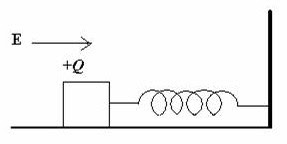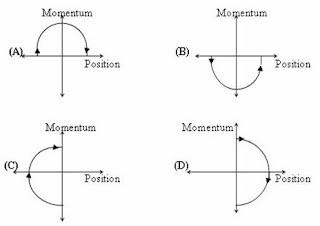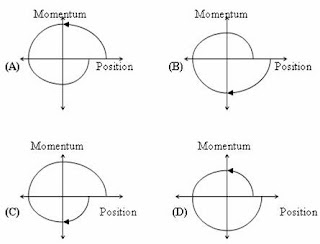## Thursday, April 28, 2011

### IIT-JEE 2011 Questions (MCQ) on Simple Harmonic Motion

“Do not worry about your problems with mathematics, I assure you mine are far greater.”

– Albert Einstein

The following two questions on simple harmonic motion were included in the IIT-JEE 2011 question paper. Both questions are simple for those who have mastered the fundamental principles:(1) A wooden block performs SHM on a frictionless surface with frequency, ν0. The block carries a charge +Q on its surface. If now a uniform electric field E is switched on as shown, then the SHM of the block will be

(A) of the same frequency and with shifted mean position

(B) of the same frequency and with the same mean position

(C) of changed frequency and with shifted mean position

(D) of changed frequency and with the same mean position

When the uniform electric field is switched on, the positively charged block experiences a constant force in the direction of the electric field and so the mean position of the block is shifted. But the frequency (ν) of oscillation of the block is unchanged since it depends only on the mass m of the block and the force constant k of the spring, in accordance with the relation

ν = (1/2π) [√(k/m)]

The correct option is (A).

(2) A point mass is subjected to two simultaneous sinusoidal displacements in x-direction, x1(t) = A sin ωt and x2(t) = A sin(ωt + 2π/3). Adding a third sinusoidal displacement x3(t) = B sin(ωt + φ) brings the mass to a complete rest. The value of B and φ are

(A) √2 A, 3π/4

(B) A, 4π/3

(C) √3 A, 5π/6

(D) A, π/3

The particle in the above question is subjected to two simultaneous simple harmonic motions (SHM) of the same frequency and amplitude in the same direction. the resultant motion of the particle is simple harmonic as is evident by adding x1(t) and x2(t):

x1(t) + x2(t) = A sin ωt + A sin(ωt + 2π/3) = A sin(ωt +π/3)

The amplitude of the resultant simple harmonic motion is A itself but the initial phase of the motion is now π/3.

As the particle remains at rest on adding the third simple harmonic motion, x3(t) = B sin(ωt + φ) the amplitude (B) of the third SHM must be A itself, but it must be 180º (or, π radian) out of phase. In other words, the initial phase (φ) of the third simple harmonic motion must be π/3 + π = 4π/3

The correct option is (B).

You can find a useful post on simple harmonic motion here.

## Friday, April 15, 2011

### IIT-JEE 2011 – Paragraph Type Multiple Choice (Single Answer) Questions Involving Phase Space Diagrams

“The earth provides enough to satisfy every man’s needs, but not every man’s greed.”

Mahatma Gandhi

The practice of asking two or three multiple choice questions (often single answer type) based on a given paragraph, is resorted to in many entrance examinations. The following three questions[(1), (2) and (3)] which appeared in IIT-JEE 2011 question paper are relatively simple:

Paragraph for questions (1), (2) and (3)

Phase space diagrams are useful tools in analyzing all kinds of dynamical problems. They are especially useful in studying the changes in motion as initial position and momentum are changed. Here we consider some simple dynamical systems in one–dimension. For such system, phase space is a plane is which position is plotted along horizontal axis and momentum is plotted along vertical axis. The phase space diagram is x (t) vs p(t) curve in this plane. The arrow on the curve indicates the time flow. For example, the phase space diagram for a particle moving with constant velocity is a straight line as shown in the figure. We use the sign convention in which position or momentum upwards (or to right) is positive and downwards (or to left) is negative.

(1) The phase space diagram for a ball thrown vertically up from the ground isThe arrow on the diagram shows the flow of time. Initially the ball is on the ground and its velocity and momentum have maximum positive values (since it moves upwards). At the highest point of its trajectory the displacement of the ball has maximum positive value but its momentum is zero. Finally when the ball just hits the ground, its displacement is zero but its momentum has maximum negative value(since it is moving downwards).

The correct option is obviously (D).(2) The phase space diagram for simple harmonic motion is a circle centered at the origin. In the figure, the two circles represent the same oscillator but for different initial conditions, and E1 and E2 are the total mechanical energies respectively. Then

(A) E1 = √2 E2

(B) E1 = 2 E2

(C) E1 = 4 E2

(D) E1 = 16 E2

When the total energy of the simple harmonic motion (SHM) is E1, the amplitude is 2a and when the total energy is E2, the amplitude is a, as is evident from the phase space diagrams. The total energy E of a particle of mass m in simple harmonic motion of amplitude A and angular frequency ω is given by

E = ½ 2A2

So when the amplitude is doubled, the total energy is quadrupled.

Thus E1 = 4 E2 as given in option (C).

[The phase space diagram given in the above question represents an undamped simple harmonic motion since respective amplitudes appropriate to the initial conditions remain constant. If the simple harmonic motion is a damped one, the curve will be a spiral, proceeding inwards].(3) Consider the spring-mass system, with the mass submerged in water, as shown in the figure. The phase space diagram for one cycle of this system isThe correct option is (B) on account of the following reasons:

(i) The amplitude of oscillations must decrease with time [which is not the case in diagrams (C) and (D)] as the viscous forces in water damps the motion of the system.

(ii) When the displacement is maximum positive value (corresponding to maximum positive position co-ordinate), the momentum is zero and is going to increase in magnitude in the negative direction [unlike in the case of diagram (A)].

## Saturday, April 09, 2011

### All India Pre-Medical/Pre-Dental Entrance Examination (AIPMT) 2011 Questions on Magnetic Force

“I have no special talents. I am only passionately curious.”

– Albert Einstein

Two questions involving magnetic force on current carrying conductors and moving charges were included in the AIPMT 2011 (Preliminary) question paper. Here are those questions with solution:(1) A current carrying closed loop in the form of a right angled isosceles triangle ABC is placed in a uniform magnetic field acting along AB. If the magnetic force on the arm BC is F, the force on the arm AC is

(1) √2 F

(2) √2 F

(3) F

(4) F

Since the current loop is placed in a uniform magnetic field, the net force acting on the loop is zero.

[Note that a current loop in a uniform magnetic field will experience a torque but no net force. If the magnetic field is non-uniform there will be a torque as well as a net force].

Since the arm AB of the loop is parallel to the magnetic field, the magnetic force on AB is zero. Since the magnetic force on the arm BC is F (as given in the question), the magnetic force on the arm Ac must be F (so as to make the net force on the loop zero).

(2) A uniform electric field and a uniform magnetic field are acting along the same direction in a certain region. If an electron is projected in the region such that its velocity is pointed along the direction of the fields, then the electron

(1) will turn towards left of the direction of motion

(2) will turn towards right of the direction of motion

(3) speed will decrease

(4) speed will increase

The magnetic force will be zero since the electron is projected parallel to the field. The electric field will exert a force opposite to the direction of motion of the electron and hence its speed will decrease [Option (3)].

## Friday, April 01, 2011

### All India Pre-Medical/Pre-Dental Entrance Examination (Preliminary) 2010 (AIPMT 2010) Multiple Choice Questions on Centre of Mass

The following questions (MCQ) on centre of mass were included in the AIPMT 2010 question paper:

(1) A man of 50 kg mass is standing in a gravity free space at a height of 10 m above the floor. He throws a stone of 0.5 kg mass downwards with a speed 2 ms–1. When the stone reaches the floor, the distance of the man above the floor will be

(1) 20 m

(2) 9.9 m

(3) 10.1 m

(4) 10 m

You can work out this question either by using the concept of centre of mass or by applying the law of conservation of momentum.

The position of the centre of mass of the man-stone system will be unchanged as there are no external forces. If x is the distance of the man from the centre of mass when the stone reaches the floor, we have

50×x = 0.5×10 from which x = 0.1 m

As the centre of mass of the system is initially at a distance of 10 m above the floor, the distance of the man when the stone reaches the floor will be 10 + x = 10.1 m.

[You can apply the law of conservation of momentum and obtain the ‘recoil speed’ v of the man from the equation, 50×v = 0.5×2, from which v = 0.02 ms–1.

The time taken by the stone to reach the floor is 10/2 = 5 s. During this time the man will move up through a distance 0.02×5 = 0.1 m so that when the stone reaches the floor, the man will be at a distance 10 + 0.1 = 10.1 m above the floor].

(2) Two particles which are initially at rest move towards each other under the action of their internal attraction. If their speeds are v and 2v at any instant, then the speed of centre of mass of the system will be

(1) v

(2) 2 v

(3) zero

(4) 1.5 v

Since the particles are initially at rest, the speed of the centre of mass of the system is zero. Since there are no external forces on the system, the centre of mass will continue to be at rest even though the particles are moving under the action of their internal attraction. Therefore the correct option is (3).

By trying a search for ‘centre of mass’ using the search box provided on this page, you can access all posts on centre of mass on this site.

You will find some additional posts on centre of mass at AP Physics Resources.